GMAT Math : DSQ: Calculating the volume of a tetrahedron

Example Questions

1 3 Next →

Example Question #189 : Geometry

Pyramid 1 in three-dimensional coordinate space has as its base the square with vertices at the origin,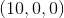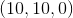, and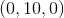, and its apex at the point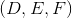; Pyramid 2 has as its base the square with vertices at the origin,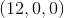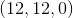, and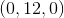, and its apex at the point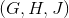. All six variables represent positive quantities. Which pyramid has the greater volume?

Statement 1: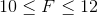and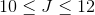Statement 2: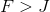Statement 1 ALONE is sufficient to answer the question, but Statement 2 ALONE is NOT sufficient to answer the question.

BOTH statements TOGETHER are insufficient to answer the question.

EITHER statement ALONE is sufficient to answer the question.

BOTH statements TOGETHER are sufficient to answer the question, but NEITHER statement ALONE is sufficient to answer the question.

Statement 2 ALONE is sufficient to answer the question, but Statement 1 ALONE is NOT sufficient to answer the question.

BOTH statements TOGETHER are insufficient to answer the question.

Explanation:

The volume of a pyramid is one third the product of its height and the area its base.

Pyramid 1 is shown below: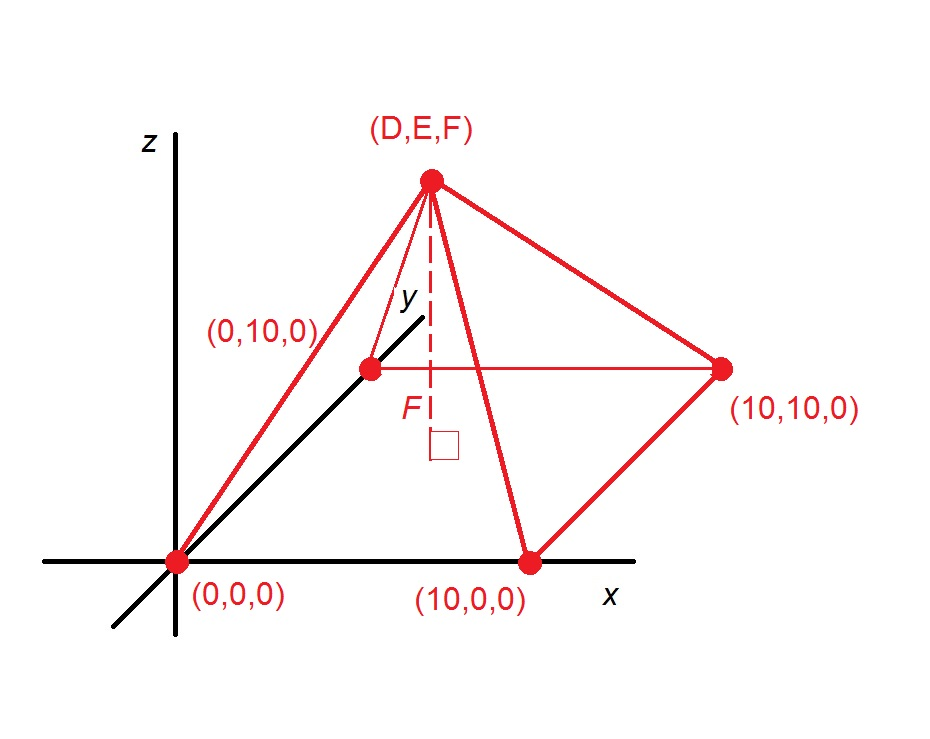The base of the pyramid is on the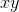-plane, so the height of the pyramid  is the perpendicular distance from apexto this plane; this is the-coordinate,. The base of the pyramid is a square of sidelength 10, so its area is the square of 10, or 100. This makes the volume of Pyramid 1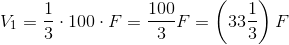Similarly, the volume of Pyramid 2 is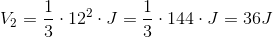Therefore, the problem asks us to determine which of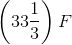and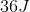is the greater.

We show that the two statements together provide insufficient information by examining two scenarios.

Case 1: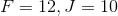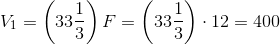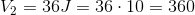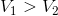Case 2: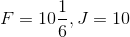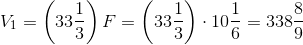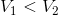Each case fits the conditions of the two statements and the main body of the question; in one case, Pyramid 1 has the greater volume and in the other case, Pyramid 2 does.

Example Question #190 : Geometry

Two right regular pyramids, Pyramid 1 and Pyramid 2, have the same height. Do the pyramids have the same volume?

Statement 1: The bases of the two pyramids have the same perimeter.

Statement 2: The base of Pyramid 1 is a hexagon; the base of Pyramid 2 is an octagon.

Statement 2 ALONE is sufficient to answer the question, but Statement 1 ALONE is NOT sufficient to answer the question.

Statement 1 ALONE is sufficient to answer the question, but Statement 2 ALONE is NOT sufficient to answer the question.

BOTH statements TOGETHER are insufficient to answer the question.

BOTH statements TOGETHER are sufficient to answer the question, but NEITHER statement ALONE is sufficient to answer the question.

EITHER statement ALONE is sufficient to answer the question.

BOTH statements TOGETHER are sufficient to answer the question, but NEITHER statement ALONE is sufficient to answer the question.

Explanation:

The volume of any pyramid is one third the product of its height and the area of its base. Since both pyramids have the same height, their volumes are equal if and only if the areas of their bases are equal as well. Also, since both pyramids are regular, their bases are regular polygons.

Statement 1 alone is insufficient. It is possible for two regular polygons to have the same perimeter but different areas. For example, an equilateral triangle with perimeter 60 has sidelength 20 and, consequently, area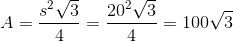A square with perimeter 60 has sidelength 15, and, consequently, area equal to the square of this, 225.

Statement 2 only gives the number of sides, and no information about their measures.

Now assume both statements are true. If the common perimeter is, then the length of one side of the base of Pyramid 1, it being a regular hexagon, is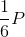, likewise, the length of one side of the base of Pyramid 2 is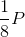. The area of each in terms ofcan be calculated, and the two can be compared.

Example Question #21 : Rectangular Solids & Cylinders

Pyramid 1 and Pyramid 2 in three-dimensional coordinate space each have the same base: the square with vertices at the origin,, and. Pyramid 1 has its fifth vertex at the point; Pyramid 2 has its fifth vertex at the point. All six variables represent positive quantities. Which pyramid, if either, has the greater volume?

Statement 1: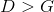Statement 2: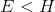BOTH statements TOGETHER are sufficient to answer the question, but NEITHER statement ALONE is sufficient to answer the question.

Statement 1 ALONE is sufficient to answer the question, but Statement 2 ALONE is NOT sufficient to answer the question.

EITHER statement ALONE is sufficient to answer the question.

BOTH statements TOGETHER are insufficient to answer the question.

Statement 2 ALONE is sufficient to answer the question, but Statement 1 ALONE is NOT sufficient to answer the question.

BOTH statements TOGETHER are insufficient to answer the question.

Explanation:

The volume of a pyramid is one third the product of its height and the area its base. The two pyramids have the same base, so the pyramid with the greater height will have the greater volume (and if their heights are equal, their volumes are equal).

Pyramid 1 is shown below:The base of the pyramid is on the-plane, so the height of the pyramid  is the perpendicular distance from apexto this plane; this is the-coordinate,. Pyramid 2 has the same base and apex, so its height is the-coordinate of its apex,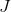Therefore, whichever is greater -or- determines which pyramid has the greater volume. However, the two statements to not give this information.

Example Question #22 : Rectangular Solids & Cylinders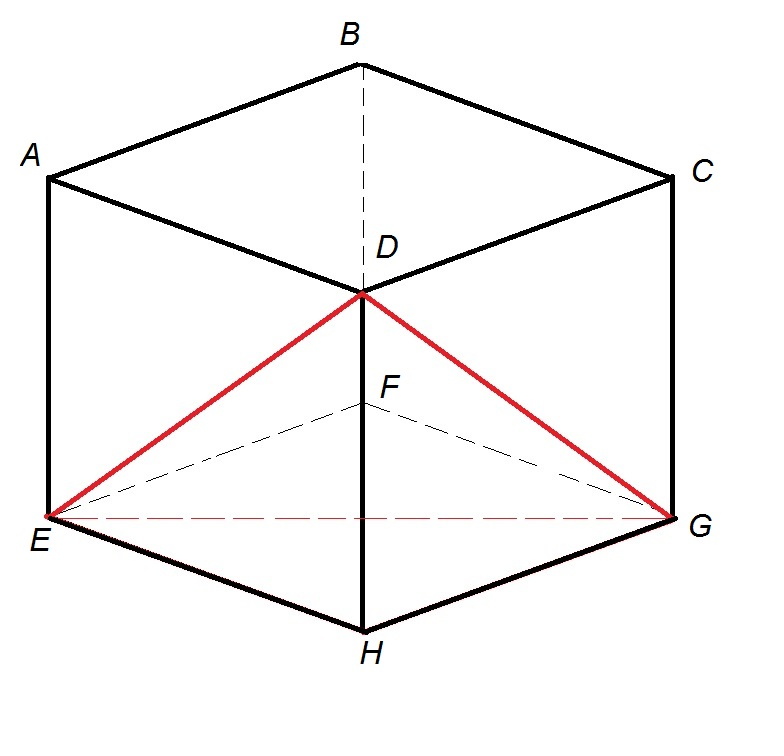In the above diagram, a tetrahedron—a triangular pyramid—with vertices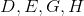is shown inside a cube. Give the volume of the tetrahedron.

Statement 1: The cube can be inscribed inside a sphere with volume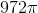.

Statement 2: A sphere with surface area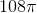can be inscribed inside the cube.

Statement 2 ALONE is sufficient to answer the question, but Statement 1 ALONE is NOT sufficient to answer the question.

Statement 1 ALONE is sufficient to answer the question, but Statement 2 ALONE is NOT sufficient to answer the question.

EITHER statement ALONE is sufficient to answer the question.

BOTH statements TOGETHER are insufficient to answer the question.

BOTH statements TOGETHER are sufficient to answer the question, but NEITHER statement ALONE is sufficient to answer the question.

EITHER statement ALONE is sufficient to answer the question.

Explanation:

The volume of the pyramid is one third the product of height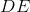and the area of its base, which in turn, since here it is a right triangle, is half the product of the lengths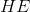and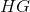of its legs. Since the prism in the figure is a cube, the three lengths are equal, so we can set each to. The volume of the pyramid is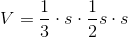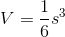Therefore, knowing the length of one edge of the cube is sufficient to determine the volume of the pyramid.

Assume Statement 1 alone. If the volume of the circumscribing sphere is known to be, the radius can be calculated as follows: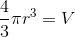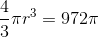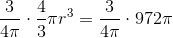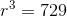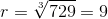The diameter, which is twice this, or 18, is the length of a diagonal of the cube. By the three-dimensional extension of the Pythagorean Theorem, the relationship of this length to the side length of the cube is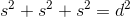, or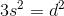so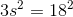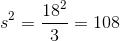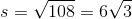,

Assume Statement 2 alone. If the surface area of the inscribed sphere is known to be, then its radius can be calculated as follows: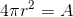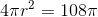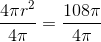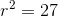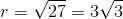.

The diameter of the inscribed sphere, which is twice this, or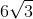, is equal to the lengthof one edge of the cube.

Either statement alone gives us the length of one side of the cube, which is enough to allow the volume of the pyramid to be calculated.

1 3 Next →

Tired of practice problems?

Try live online GMAT prep today.